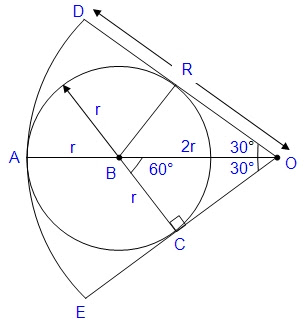## Sunday, July 11, 2010

### Secondary 4 Circle and trigonometry (Hey Math)

From: Hey Math! assignment (E-Maths), July 2010, Question #7, Circle and trigonometry

An arc which subtends and angle of 60° at O is shown below. Find the ratio of r : R.Select the correct answer from the following choices.
i) 1 : 2
ii) 1 : √2
iii) 1 : √3
iv) 1 : 3

Solutions:

With reference to the following diagram;The radius ABO passed through the center of the circle ARC and bisects the angle EOD.

Triangle BCO is a right angled triangle with angles 30°, 60° and 90°.

By trigonometry,
if BC = r, then
BO = 2r.

Therefore radius of the arc DAE = AB + BO = 3r

Given that the radius of the arc DAE = R,

That is, R = 3r

Therefore the ratio of r : R is 1 : 3.